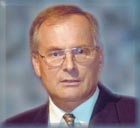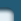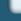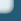Research Interests

Web design by Alexei Kuntsevich### Research interests

Instead of a list of keywords characterising my research interests I give a short description of these in their approximate historical development.Ā It is clear that the fields are more or less interconnected. Papers for each of the research areas can be collected from the list of publications using the keywords given in brackets.

At the beginning of my academic career nuclear reactor kinetics was one of the areas of interest. The corresponding papers are mainly concerned with stability properties of so called point kinetic models. The approach used was mainly Liapunov's direct method. Naturally, at the same time stability theory was another research area. Most contributions to this area are concerned with Liapunov's direct method. Besides the papers on nuclear reactor stability there are investigations concerning the invariance of limit sets for infinite delay equations which is the basis for the so called invariance principle by Barbashin-Krasovskij-LaSalle. Also problems related to stability in the first approximation were considered, the difference to the usual problem being that each of the coordinates of the perturbation satisfies a sector condition. For linear autonomous delay equations of retarded type an elementary divisor theory was established. Whereas the two areas discussed up to now are presently not active any more, a third area – delay equations of various types – originated at the same time and is still of interest to me. Besides stability problems, very soon the so called degeneracy problem for retarded delay equations was in the centre of interest. In order to obtain algebraic criteria results on 'normal' forms for polynomial matrices under similarity transforms had to be established. At about the same time the development of numerical algorithms for approximating solutions and for solving linear-quadratic control problems became a very active area of research. The approach was based on abstract approximation results for strongly continuous semigroups of transformations (Trotter-Kato theorem). For concrete algorithms splines or orthogonal polynomials have been used. Besides delay equations of retarded type also equations of neutral type and equations with infinite resp. state dependent delays were considered. Of particular interest was preservation of spectral properties by the approximating ordinary differential equations. Furthermore a systematic treatment of autonomous linear delay equations of retarded and of neutral type based on Laplace-transform resp. Laplace-Stieltjes-transforms were presented. An interesting class of equations investigated using the semigroup approach are delay equations of neutral type with singular difference operator. Contributions to the basic theory of such equations have been obtained. The approach used for approximation of delay equations was also carried over to other types of equations: age and size structured population models, partial differential equations. In this context also variational formulations of the Trotter-Kato theorem were proved. It was also shown that the Trotter-Kato theorem can provide rate estimates for approximation schemes. Semigroups were involved in almost all papers concerned with approximation of dynamical systems. This was not only restricted to linear problems. There are a few approximation results on non-linear delay equations using corresponding abstract results on semigroups of non-linear transformations. A differentĀ approach was to consider the non-linearity in the delay equation as a perturbation of the zero right-hand side. It was for instance proved that for certain non-linear delay equations the approximating equations preserve Hopf bifurcations. From the interest in semigroups emerged a growing interest in the theory of abstract evolution equations with time dependent quasi-dissipative time-dependent operators. A book project will be completed shortly. Objective is a systematic presentation of the approach by Kobayashi-Oharu. Recently dynamical reconstruction of state, input and output variables – also for delay systems – was of special interest. Emphasis is on robust algorithms.

Always of interest were concrete applications, which results in a considerable amount of research efforts concerning mathematical modelling. The interest in population models with size or age structure was caused by modelling efforts for fish populations. Motivated by this concrete modelling projects were contributions to the theory of populations which show a dispersion of growth rates among individuals. Since many years cardiovascular modelling is in the centre of my research in applications. This modelling process is concerned with basic regulatory mechanisms in the cardiovascular system under an ergometric workload. Recently the model has been extended in order to include also the respiratory system. Applications to congestive hard failure and to the transition from the state of awakeness to non-REM sleep are considered. Another area of research was concerned with some optimisation problems in connection with models for the dialysis process .